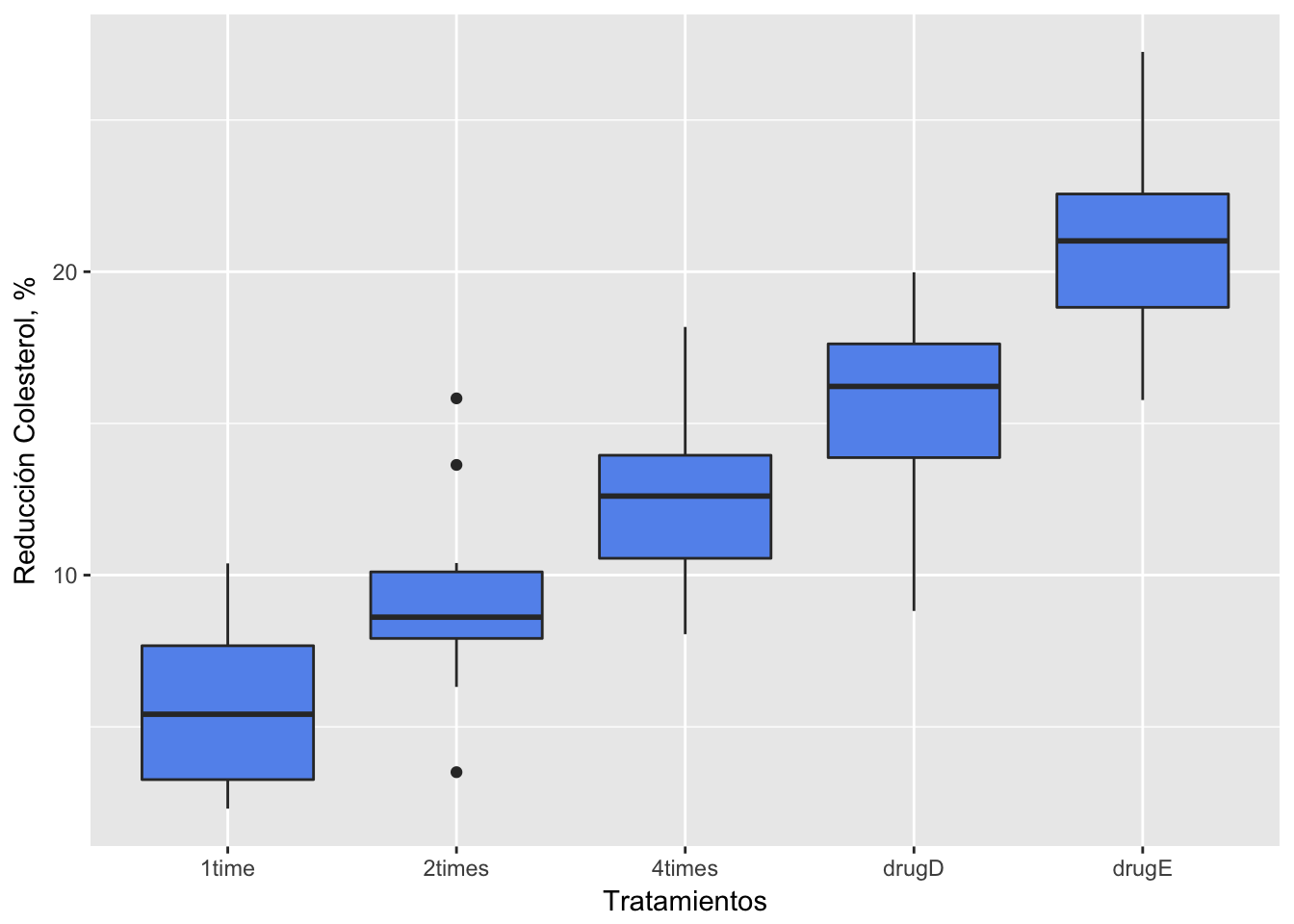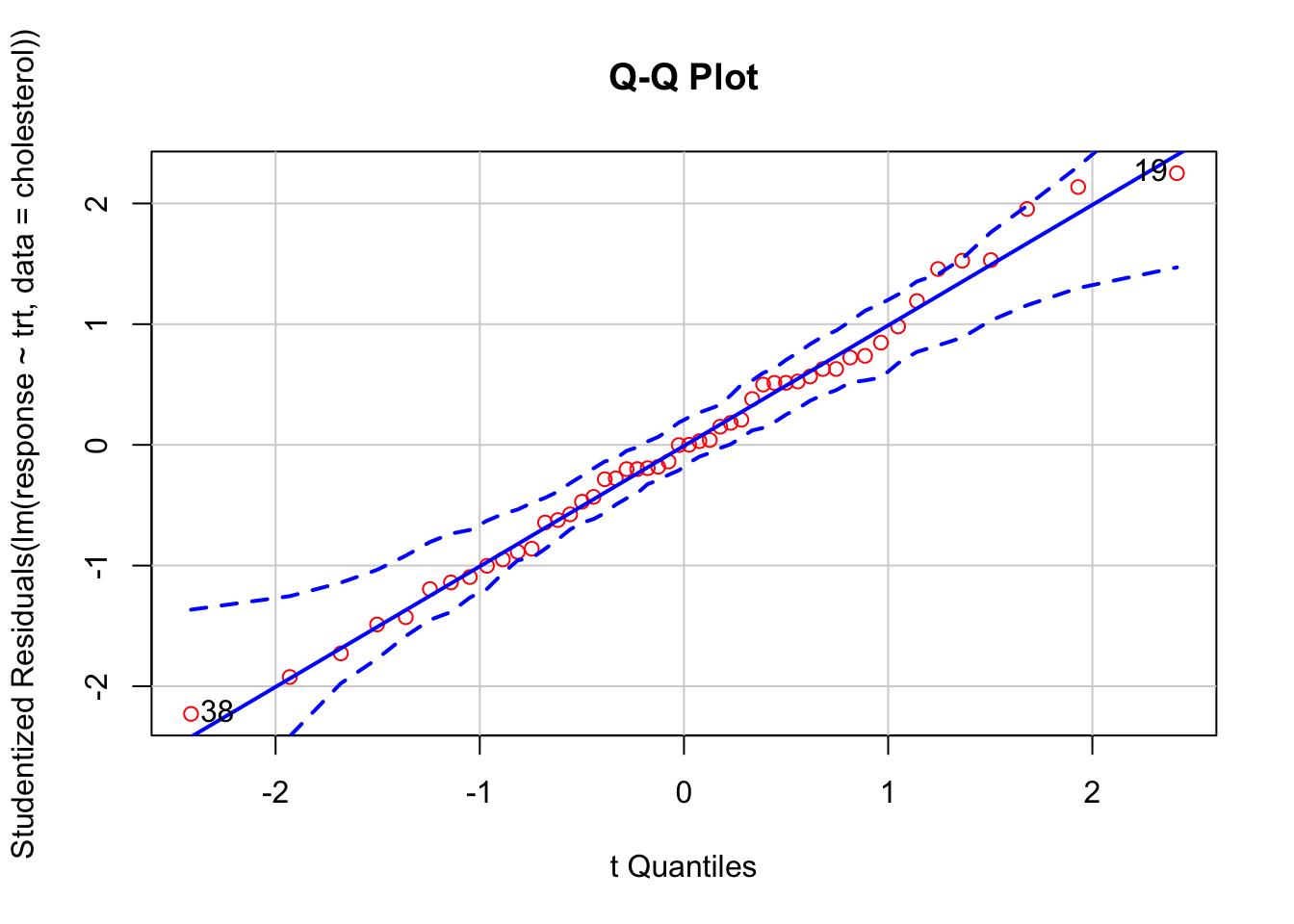``````knitr::opts_chunk\$set(echo = TRUE)
library(multcomp)``````
``## Loading required package: mvtnorm``
``## Loading required package: survival``
``## Loading required package: TH.data``
``## Loading required package: MASS``
``````##
## Attaching package: 'TH.data'``````
``````## The following object is masked from 'package:MASS':
##
##     geyser``````
``library(car)``
``## Loading required package: carData``

### Analysis of variance (ANOVA)

Previously we considered regression models for predicting a quantitative response variable from quantitative predictor variables. But we can include nominal or ordinal factors as predictors as well. When factors are included as explanatory variables, our focus usually shifts from prediction to understanding group differences, and the methodology is referred to as analysis of variance (ANOVA). ANOVA methodology is used to analyze a wide variety of experimental and quasi-experimental designs.

#### One-way ANOVA

In the following example, fifty patients received one of five cholesterol-reducing drug regimens (trt). Three of the treatment conditions involved the same drug administered as 20 mg once per day (1time), 10mg twice per day (2times), or 5 mg four times per day (4times). The two remaining conditions (drugD and drugE) represented competing drugs. Which drug regimen produced the greatest cholesterol reduction (response)?

##### ANOVA visualization using ggplot2

We are going to use box-plots to observe differences between treatments, using ggplot2.

``````library(multcomp)
data(cholesterol)
cholesterol``````
``````library(ggplot2)
ggplot(cholesterol, aes(x=trt, y=response)) + geom_boxplot(fill="cornflowerblue") +
labs(x = "Tratamientos", y = "Reducción Colesterol, %")``````In a one-way ANOVA, we are interested in comparing the dependent variable means of two or more groups defined by a categorical grouping factor.

``````attach(cholesterol)
table(trt)``````
``````## trt
##  1time 2times 4times  drugD  drugE
##     10     10     10     10     10``````
``aggregate(response, by=list(trt), FUN=mean) ``
``aggregate(response, by=list(trt), FUN=sd)   ``
``````fit <- aov(response ~ trt)
summary(fit)``````
``````##             Df Sum Sq Mean Sq F value   Pr(>F)
## trt          4 1351.4   337.8   32.43 9.82e-13 ***
## Residuals   45  468.8    10.4
## ---
## Signif. codes:  0 '***' 0.001 '**' 0.01 '*' 0.05 '.' 0.1 ' ' 1``````
``detach(cholesterol)``

The results of the analysis indicate that there are significant differential effects of the treatments to reduce cholesterol, this is because the probability of a real no-difference (type I error) is very low (Pr(>F) = 9.82e-13).

But, wait! To apply the procedure above, there are a couple of requirements from the data:

• data must have a normal distribution
• equality of variance between the groups

First will see if the response variable has a normal distribution:

``````library(car)
qqPlot(lm(response ~ trt, data=cholesterol),
simulate=TRUE, main="Q-Q Plot", labels=FALSE, col = "red")````````##  19 38``

The data falls within the 95% confidence envelope, suggesting that the normality assumption has been met fairly well.

Now we will test homogeneity of variance, using the Bartlett’s test:

``bartlett.test(response ~ trt, data=cholesterol)``
``````##
##  Bartlett test of homogeneity of variances
##
## data:  response by trt
## Bartlett's K-squared = 0.57975, df = 4, p-value = 0.9653``````

Bartlett’s test indicates that the variances in the five groups do not differ significantly (p = 0.97).

##### Pairwise comparisson

The ANOVA F test for treatment tells you that the five drug regimens are not equally effective, but it does not tell you which treatments differ from one another. You can use a multiple comparison procedure to answer this question. For example, the TukeyHSD() function provides a test of all pairwise differences between group means, as shown next.

``TukeyHSD(fit)``
``````##   Tukey multiple comparisons of means
##     95% family-wise confidence level
##
## Fit: aov(formula = response ~ trt)
##
## \$trt
##                   diff        lwr       upr     p adj
## 2times-1time   3.44300 -0.6582817  7.544282 0.1380949
## 4times-1time   6.59281  2.4915283 10.694092 0.0003542
## drugD-1time    9.57920  5.4779183 13.680482 0.0000003
## drugE-1time   15.16555 11.0642683 19.266832 0.0000000
## 4times-2times  3.14981 -0.9514717  7.251092 0.2050382
## drugD-2times   6.13620  2.0349183 10.237482 0.0009611
## drugE-2times  11.72255  7.6212683 15.823832 0.0000000
## drugD-4times   2.98639 -1.1148917  7.087672 0.2512446
## drugE-4times   8.57274  4.4714583 12.674022 0.0000037
## drugE-drugD    5.58635  1.4850683  9.687632 0.0030633``````
``````par(las=2)
par(mar=c(5,8,4,2))
plot(TukeyHSD(fit))``````